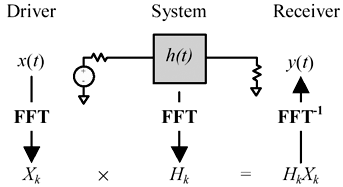# Going Nonlinear

## Article first published in EDN Magazine , May 16, 2002

SPICE is grand for solving circuits that have complicated topologies or include nonlinear elements. If, however, you are working with a simple, linear circuit, you might question whether SPICE is best.

For example, suppose you are designing a 2.5-Gbps serial-communications channel terminated at both ends. Your source- terminated , 100- W driver is a highly linear creature. So is your load. Simulations of this circuit therefore produce the same output whether you use a linear-analysis tool or SPICE.

The linear-analysis method is the same as the frequency-domain method (Figure 4.1). To perform linear analysis, you first transform your excitation waveform x ( t ) into the frequency domain. You accomplish the transformation using a canned FFT algorithm. It produces a vector of frequency-domain values, X k , sampled on a very dense grid of frequencies. Next, you compute the frequency response of the communication channel. If your system comprises a transmission line with frequency-varying values of R, L, G , and C combined with some terminators, you can directly write down an expression for the frequency response.  Then, for every frequency on the dense grid, multiply X k by the frequency response of the communication channel, H k , and apply an inverse FFT to convert the resulting spectrum back into a time-domain waveform. The FFT method handles reflections at the source; reflections at the load; impedance mismatches within the communications channel; linear filters at the transmitter, receiver, or both; connector effects; and extended data patterns.

Figure 4.1. Canonical form of linear systems analysis.The FFT shines as an efficient computational tool for long transmission channels. Whenever the bulk transport delay of the channel exceeds the risetime of the driver, a time-step simulator must break the channel into many tiny sections, independently simulating each section. A simulation with N channel sections and M time steps requires N · M separate simulation activities. A frequency-based simulation, on the other hand, computes the performance of the communication channel as a whole, proceeding with only one computation (albeit a very complex one) for each frequency on the dense grid. When the number of simulated cable sections in the time-step method becomes large, the FFT-based approach computes much more rapidly .

The FFT shines as an efficient computational tool for long transmission channels.

The FFT method is far from perfect. It assumes the underlying system has a simple topology and is both linear and time-invariant . That means, for example, that you cannot analyze a diode termination (a nonlinear circuit) using the FFT method. Fortunately, most transceivers on long communication channels adhere to the linear-time-invariant assumption, so FFT-based simulation of serial-communications links usually delivers excellent results.

Next time you face a challenging high-speed serial-communications problem, consider the advantages of frequency-based simulation before you go totally nonlinear.

 See Chapter 3, and Appendix C.

POINTS TO REMEMBER

• Frequency-domain simulation gives you incredible control over the exact form of frequency-dependent losses, like the skin effect and dielectric-loss effect.
• Frequency-domain simulators may be easily programmed in any software spreadsheet application (like MatLab, Mathematica, or MathCad), giving you control over every aspect of the simulation, including searching for optimum and worst-case parameter values.
• Frequency-domain simulation applies only to linear systems.High-Speed Signal Propagation[c] Advanced Black Magic
ISBN: 013084408X
EAN: N/A
Year: 2005
Pages: 163